DraftKings Championship Odds
+220
2.2 to 1Nets
26.3% implied probability

+500
5 to 1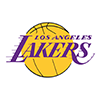Lakers
14.0% implied probability

+800
8 to 1Bucks
9.3% implied probability

+900
9 to 1Warriors
8.4% implied probability

+1300
13 to 1Jazz
6.0% implied probability

+1700
17 to 1Clippers
4.7% implied probability

No changes have been recorded yet.
+1700
17 to 1Suns
4.7% implied probability

+2200
22 to 1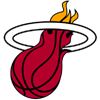Heat
3.7% implied probability

+2200
22 to 176ers
3.7% implied probability

+2200
22 to 1Nuggets
3.7% implied probability

+3000
30 to 1Mavericks
2.7% implied probability

+3500
35 to 1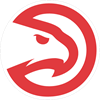Hawks
2.3% implied probability

No changes have been recorded yet.
+4000
40 to 1Celtics
2.1% implied probability

No changes have been recorded yet.
+5000
50 to 1Bulls
1.6% implied probability

+7000
70 to 1Grizzlies
1.2% implied probability

+8000
80 to 1Trail Blazers
1.0% implied probability

No changes have been recorded yet.
+10000
100 to 1Pacers
0.8% implied probability

No changes have been recorded yet.
+10000
100 to 1Knicks
0.8% implied probability

No changes have been recorded yet.
+15000
150 to 1Pelicans
0.6% implied probability

+18000
180 to 1Hornets
0.5% implied probability

No changes have been recorded yet.
+25000
250 to 1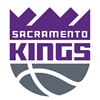Kings
0.3% implied probability

No changes have been recorded yet.
+25000
250 to 1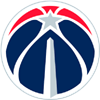Wizards
0.3% implied probability

No changes have been recorded yet.
+25000
250 to 1Timberwolves
0.3% implied probability

No changes have been recorded yet.
+30000
300 to 1Raptors
0.3% implied probability

+35000
350 to 1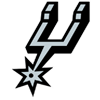Spurs
0.2% implied probability

No changes have been recorded yet.
+100000
1000 to 1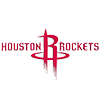Rockets
0.1% implied probability

No changes have been recorded yet.
+100000
1000 to 1Thunder
0.1% implied probability

No changes have been recorded yet.
+100000
1000 to 1Cavaliers
0.1% implied probability

No changes have been recorded yet.
+100000
1000 to 1Pistons
0.1% implied probability

No changes have been recorded yet.
+100000
1000 to 1Magic
0.1% implied probability

No changes have been recorded yet.